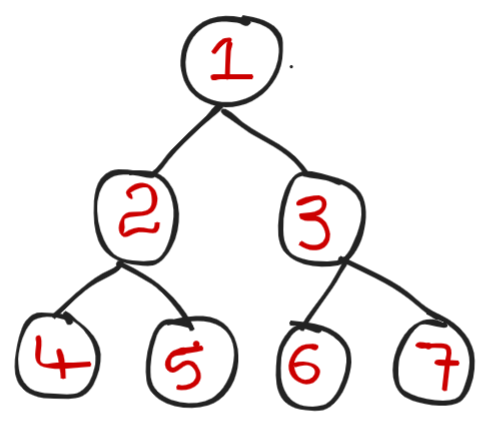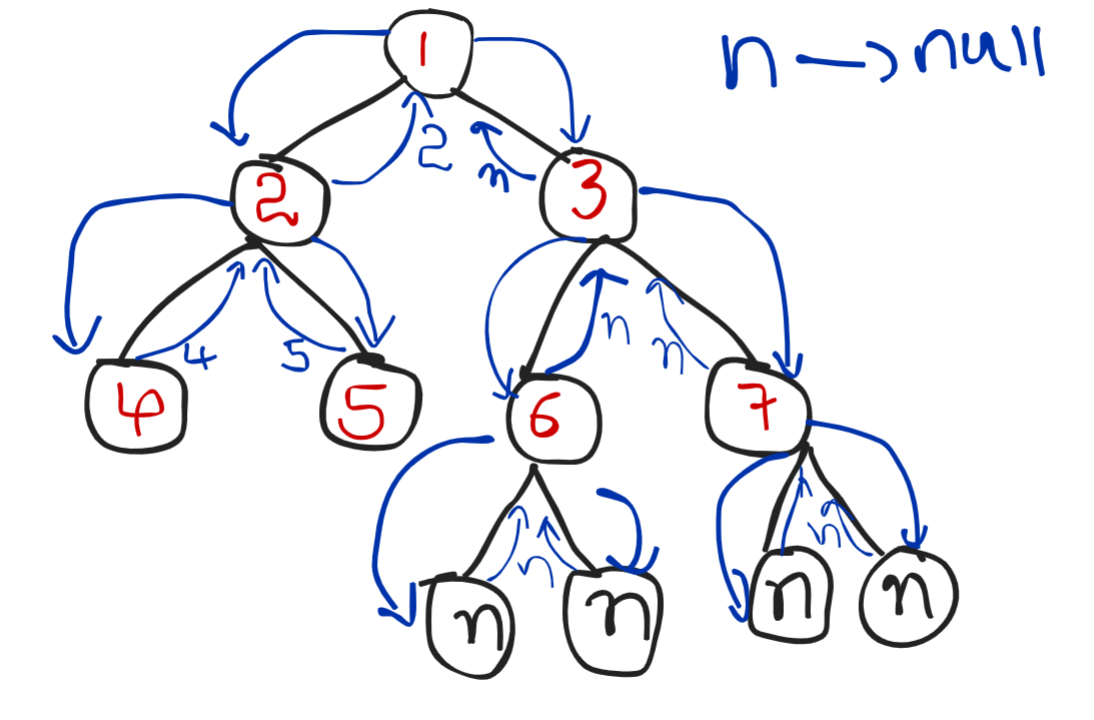# Lowest Common Ancestor for two given Nodes

Problem Statement: Given a binary tree, Find the Lowest Common Ancestor for two given Nodes (x,y).

Lowest Common Ancestor(LCA): The lowest common ancestor is defined between two nodes x and y as the lowest node in T that has both x and y as descendants (where we allow a node to be a descendant of itself.

Examples:

```Consider the following Binary TreeExample 1:

Input: x = 4 , y = 5

Output: 2

Explanation: All ancestors for 4,5 are 2,1.  But we need Lowest Common ancestor, So we will consider lowest and also common ancestor that is 2

Example 2:

Input:
x = 2 , y = 3

Output: 1

Explanation:  Lowest Common Ancestor for x,y i.e for 2,3 is 1

Example 3:

Input:
x= 6 , y = 7

Output: 3

Explanation:  Lowest Common Ancestor for x,y i.e for 6,7 is 3 ```

Solution:

Disclaimer: Don’t jump directly to the solution, try it out yourself first.

Intuition:

The very first thing we can observe from the question is that we can find the LCA of 2 given nodes from

i) Left subtree or in

ii)Right subtree, if not in both the subtrees then root will be the  LCA.

Approach:

1. If root is null or if root is x or if root is y then return root
2. Made a recursion call for both

i) Left subtree

ii)Right subtree

Because we would find LCA in the left or right subtree only.

1. If the left subtree recursive call gives a null value that means we haven’t found LCA in the left subtree, which means we found LCA on the right subtree. So we will return right.
1. If the right subtree recursive call gives null value, that means we haven’t found LCA on the right subtree, which means we found LCA on the left subtree. So we will return left .
1.  If both left & right calls give values (not null)  that means the root is the LCA.

Let’s take an example and will try to understand the approach more clearly:

### LCA of (x,y) = > (4,5) = ? (from above given example)

• Root is 1 which is not null and x,y is not equal to root, So the 1st statement in approach  will not execute.
• i) Call left subtree, While calling recursively it will find 4 and this call will return 4 to its parent

Point to Note: At present, the root is 2 ( Look at below recursion tree for better understanding)

i) Call the right subtree ( i.e right of 2), While calling recursively it will find 5  and this call will return 5 to its parent.

• Now the left recursive  call returns value (not null) i.e 4 and also the right recursive call returns value (not null) i.e 5 to its root ( at present root is 2) , and this 2 will return itself to its root i.e to 1 (main root).

Point to Note: At present, the root is 1 ( Look at below recursion tree for better understanding)

• Now, the left subtree gives a value i.e 2.
• Right recursive call will give null value .because x,y are not present in the right subtree.
• As we know if the right recursive call gives null then we return the answer which we got from the left call, So we will return 2.
•  Hence LCA of (4,5) is 2.

For a better understanding of the above example (LCA OF 4,5) :Code:

## C++ Code

``````class Solution {
public:
TreeNode* lowestCommonAncestor(TreeNode* root, TreeNode* p, TreeNode* q) {
//base case
if (root == NULL || root == p || root == q) {
return root;
}
TreeNode* left = lowestCommonAncestor(root->left, p, q);
TreeNode* right = lowestCommonAncestor(root->right, p, q);

//result
if(left == NULL) {
return right;
}
else if(right == NULL) {
return left;
}
else { //both left and right are not null, we found our result
return root;
}
}
};``````

Time complexity: O(N) where n is the number of nodes.

Space complexity: O(N), auxiliary space.

## Java Code

``````class Solution {
public TreeNode lowestCommonAncestor(TreeNode root, TreeNode p, TreeNode q) {
//base case
if (root == null || root == p || root == q) {
return root;
}
TreeNode left = lowestCommonAncestor(root.left, p, q);
TreeNode right = lowestCommonAncestor(root.right, p, q);

//result
if(left == null) {
return right;
}
else if(right == null) {
return left;
}
else { //both left and right are not null, we found our result
return root;
}
}
}``````

Time complexity: O(N) where n is the number of nodes.

Space complexity: O(N), auxiliary space.

Special thanks to Sai bargav Nellepalli for contributing to this article on takeUforward. If you also wish to share your knowledge with the takeUforward fam, please check out this article